﻿ Molecular bond, Huckel method is useless

# "Spin" in molecule ?  Forget it !

## Up, down spins form molecular bond ?

### [ Electron "spin" is unreal, too weak to form atomic bond ! ]

(Fig.1)  Unreal spin is really involved in hydrogen molecule bond ?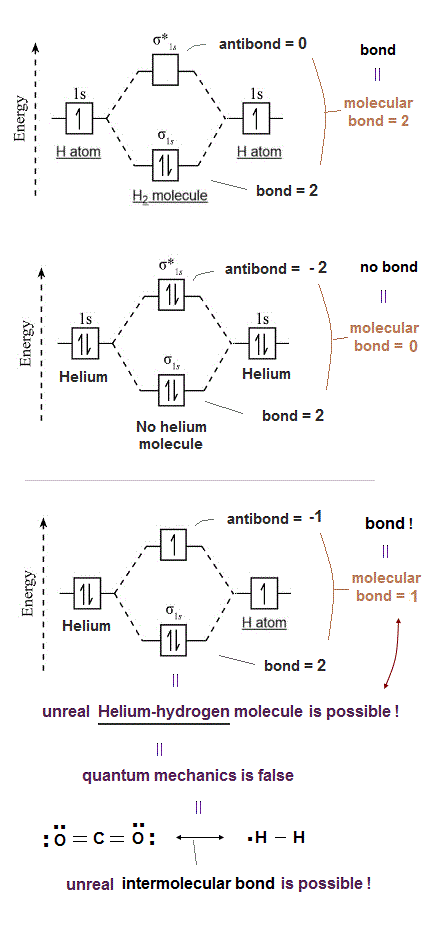Textbooks in quantum mechanics often argue that "up" and "down" spins form molecular bonds.  But in fact it's physically impossible.

Because spin-spin magnetic interaction (= only 0.001 eV ) is too weak to cause any molecular bonds (= 3-4 eV ).  So spin has nothing to do with molecular bonds !

## Electron spinning far exceeds light speed !

### [ Spinning speed of "point"-like electron is much faster than light ! ]

(Fig.2)  Point-like electron ( radius r → 0 ), rotation v → ∞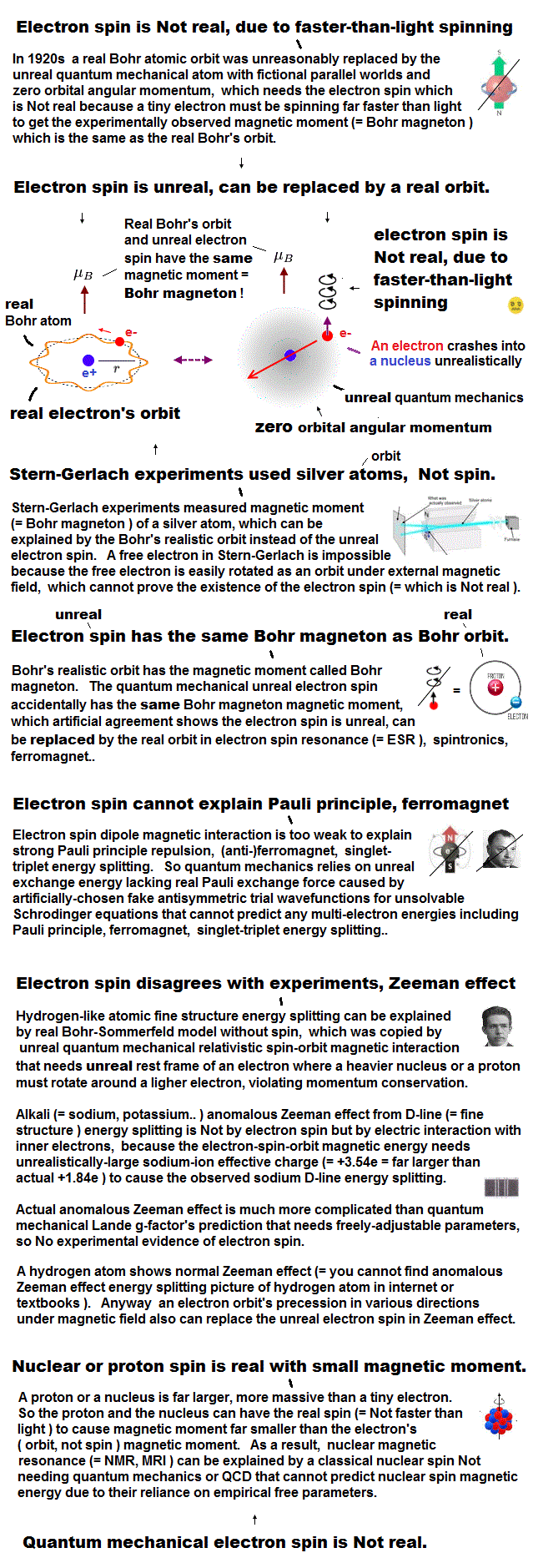Angular momentum is given by mv × r ( v = velocity, r = radius ).
Electron spin also has angular momentum 1/2ħ, they claim

The problem is an electron is very tiny, point-like.
The point-like particle means its radius r is almost zero.

So to get the angular momentum 1/2ħ, the electron spinning must far exceed light speed ( this p.5, this )

So the electron spin lacks reality.
Even Pauli ridiculed the idea of "spinning electron".

But in "s" orbital of Schrodinger's hydrogen, this electron spin is the only generator of magnetic moment.

So they had no choice but to accept this strange spin ( Not as real spinning and speed ).

## Stern-Gerlach experiment shows spin is a magnet ?

### [ Spin magnetic moment is equal to Bohr magneton (= μB ). ]

(Fig.3)  The magnitude of electron spin is just equal to Bohr magneton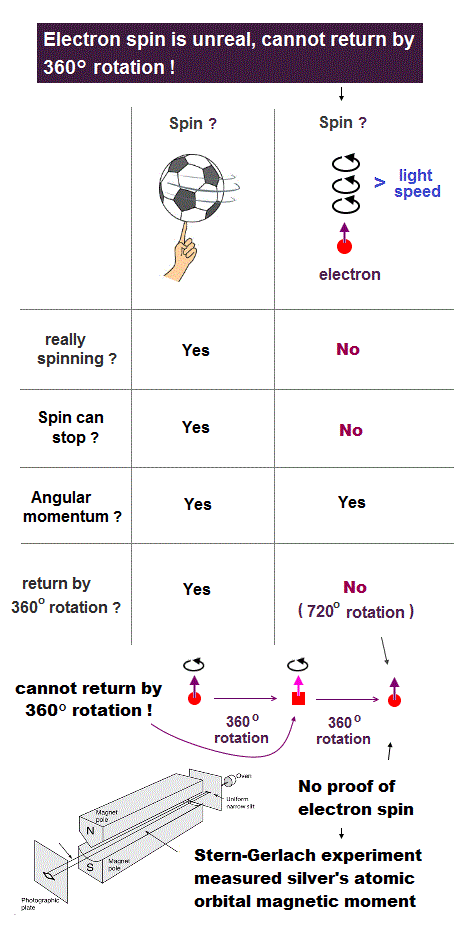In Stern-Gerlach experiment, they got some atoms through magnetic field to measure the magnitude of magnetic moment of each electron.

Surprisingly, the experiments showed that the magnitude of magnetic moment of electron spin is just equal to Bohr magneton in Bohr's classical orbit ( this p.4 )

We can safely say Stern-Gerlach experiment proved the magnetic property in Bohr's orbit ( Not unreaslitic spin ! )

## Electron spin is too weak to form molecular bond.

### [ Spin magnetic enrgy is far smaller than bond energy ! ]

(Fig.4)  Spin-spin magnetic energy (= 0.0001 eV ) vs. bond energy (= 4.5 eV )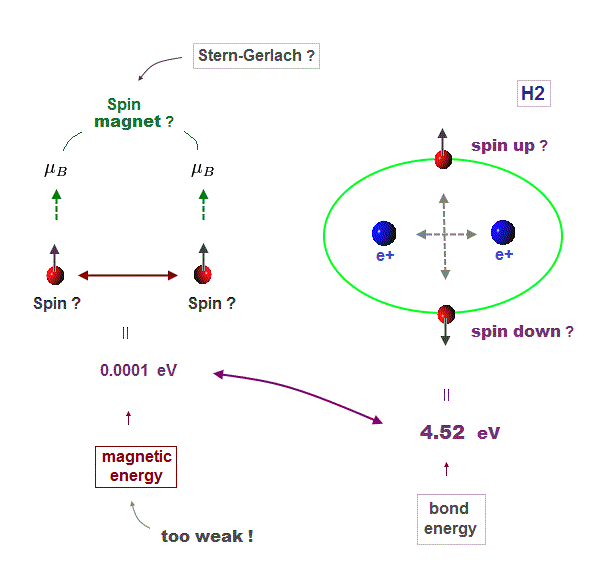You may hear spin is tiny magnet with the magnitude of Bohr magneton.
We cannot see unreal spin itself, we just measure its magnetism by experiments.

We can replace unrealistic spin by real orbital motion causing the same magnetic field.  "Spin" without other motions cannot explain actual phenomena.

Spin-spin magnetic interaction is too weak to explain actual ferromagnet.
See this p.6 this p.7.  So spin model failed from the beginning.

Hydrogen molecular bond energy is known to be as large as 4.5 eV which electrons spin's magnetic energy (= only 0.0001 eV ! ) can NEVER reach.

## Electron spin failed in describing "real" bonds.

### [ Spin is used just as "mark", irrelevant to its actual magnetic property. ]

(Fig.5)  ↓ Spin magnetism has nothing to do with molecules !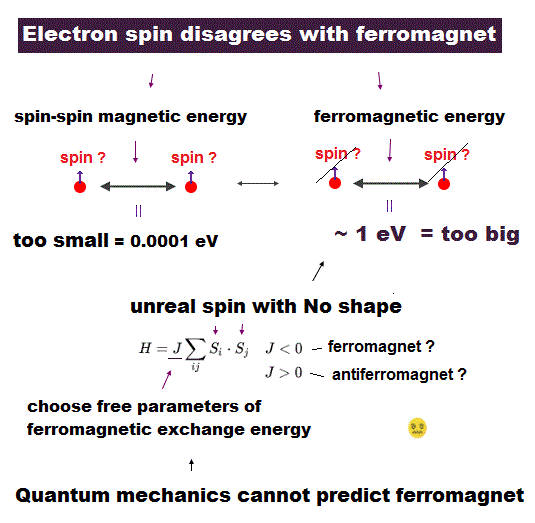Electron spin was originally defined as "tiny magnet", though its "spinning" speed far exceeds light speed c.

Its spin-spin magnetic interaction is too weak to explain actual phenomena such as Pauli exclusion principle and ferromagnetism.

So too weak spin has nothing to do with molecular bond !  In fact, spin is used only as "mark" to distinguish different spectra, ignoring its actual magnetic property.

## Spin-spin magnetic interaction never happens.

### [ The total spin-spin interaction becomes zero ! ]

(Fig.6)  Directions of magnetic field are the opposite in different places.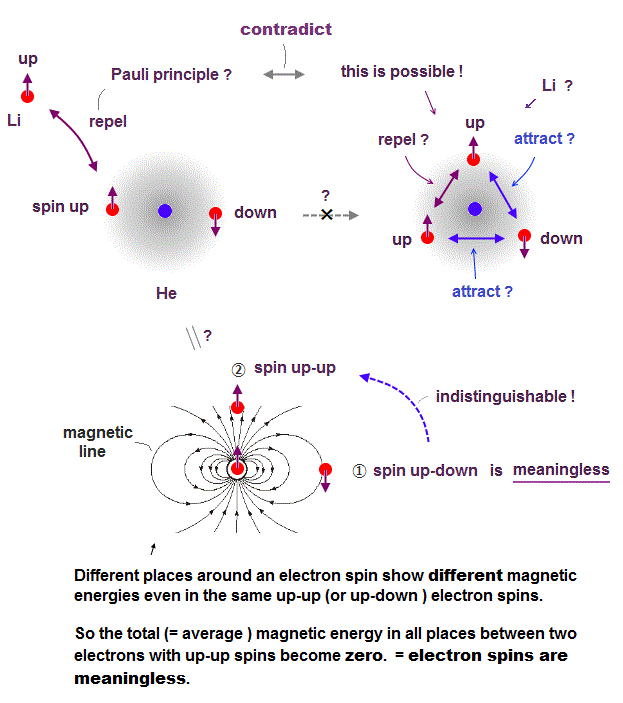Here we explain why spin-spin magnetic interaction is impossible.
Because the total magnetic energy by spin-spin is always canceled out to be zero.

Magnetic source always includes a pair of N and S poles, which generates magnetic field lines around it.

To retuen from N to S pole, the magnetic field must include two opposite directions ( upward and downward ).

Suppose two spins are always "up" and "up".  In Fig.6 left, spin direction is the same as magnetic field caused by another spin, but the opposite in Fig.6 right.

So total magnetic energy in spin-spin always becomes zero, in any spin directions.

## Pi bond in ethene is unrealistic

### [ Two electrons "repel" each other, NOT forming pi bond ! ]

(Fig.7)  Ethene carbon double bond ( H2-C=C-H2 ) is NOT "pi" bond !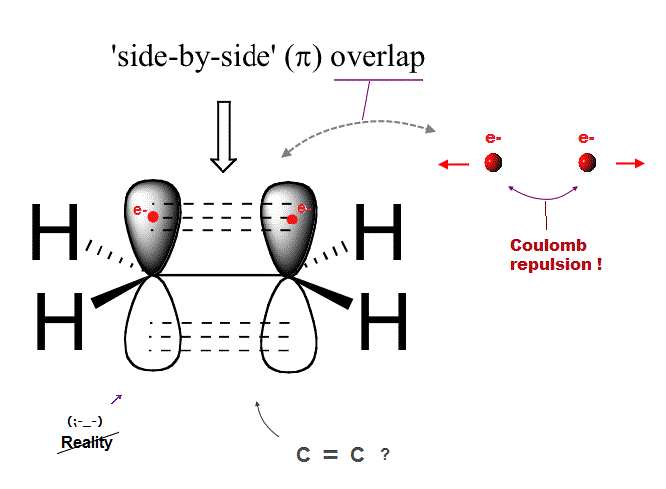Quantum mechanics claims that carbon double bond ( C=C ) is caused by pi bond between two parallel electrons.

But in fact, this "pi bond" model in quantum mechanics cannot form actual stable bond, because two negative electrons repel and avoid each other !

So orbitals of these two repulsive electrons tend to avoid lining up side by side parallel to each other !  This model doesn't describe reality.

## Quantum mechanics cannot predict double bond.

### [ Its Hückel method just artificially "adjusts" free parameters. ]

(Fig.8)  Huckel method in pi bond is "empirical" using experimental data.Huckel theory is famous as calculating method in molecular double ( pi ) bond.  But in fact, this Huckel theory invented in 1930s is useless, cannot predict any values.

Huckel method just adjusts free parameters artificially to fit experimental data.  So Huckel is empirical, NOT ab-initio method.

Choosing parameters as we like means this Huckel method has NO ability to predict any values useful for us.  Quantum mechanics remains useless.

## Schrödinger equation is useless in multi-electrons.

### [ There is NO exact solution in multi-electron atoms. ]

(Fig.9)  So they just choose "fake" trial function, NOT predicting values.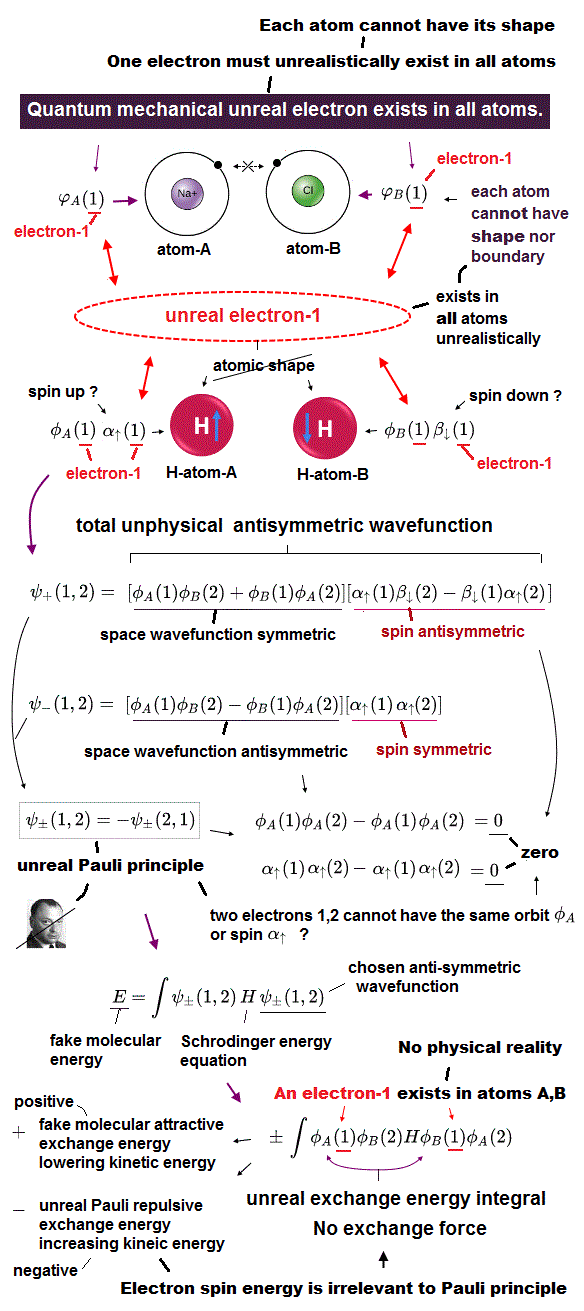Schrodinger equation cannot be solved in multi-electron atoms except for one-electron hydrogen atom.

So all physicists can do is choose approximate trial function.  The problem is there is NO restriction in choosing this trial function as candidate.

We can choose convenient form of trial wavefunction out of infinite choices.  So it's impossible to compare infinite possible trial functions giving the lowest energy.

This is the reason why Schrodinger equation is useless, has NO power to predict physical values forever, also in DFT and Nobel prize.

## Why quantum mechanics is useless forever ?

### [ Choosing artificial trial function is endless. ]

(Fig.10)  Choosing approximate wavefunction from "infinite" choices !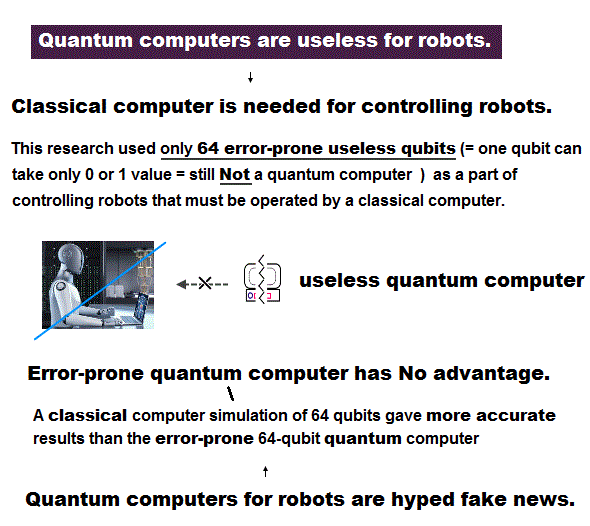Here we explain why Schrodinger equation in quantum mechanics is useless forever.  First, there is NO exact solution in multi-electron atoms.

So they just pick up some approximate wavefunction from infinite candidates, and adjusting their parameters to lower energy.

To find the lowest energy, we have to compare all possible (= infinite ) trial functions !  It's impossible ( this last ).

So after all, they just choose convenienet approximate functions ( ~ only 6 terms, this p.13 ), which is far from unknown "exact" values.

These trial wavefunctions are NOT true solution, because they cannot satisfy total energy conservation law in any positions.

## Quantum mechanics is "deadlocked"

### [ Because it sticks to useless Schrodinger equation forever. ]

(Fig.11)  No exact solution → just choose "fake" wavefunction → endless guess.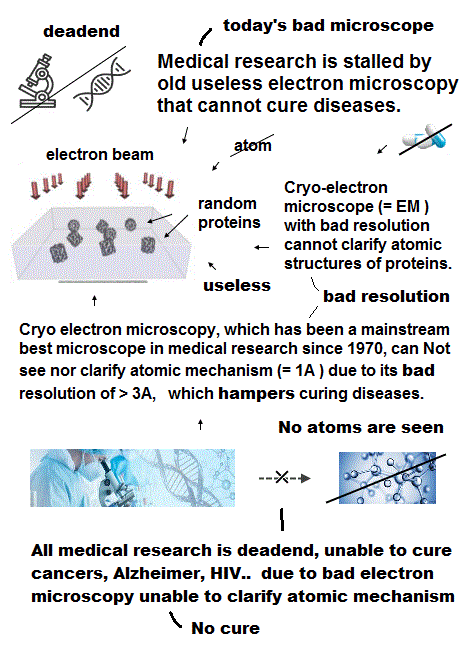Quantum mechanics is deadlocked, remains useless forever, because it cannot escape from the old process of "solving Schrodinger equation".

But Schrodinger equation in multi-electron atoms cannot be solved.  Physicists just choose approximete (= fake ) solution function out of infinite candidates.

Of course, it's impossible to try all possible forms of wavefunctions, so this quantum mechanical method is deadlocked, forever.

Furthermore, quantum trial functions have NO clear orbital picture in multi-electron atoms.

What can we do, if we can use clear orbital picture in atoms based on two postulates of Coulomb force and de Broglie wave ?

It will be much easier for us to develop and find more effective and useful new computational ways, which old quantum mechanics prevents !

## Quantum mechanics stops science !

### [ Experimental Data → fictional quasiparticle, effective mass ! ]

(Fig.12)  We must use experimental data to predict "real concept" !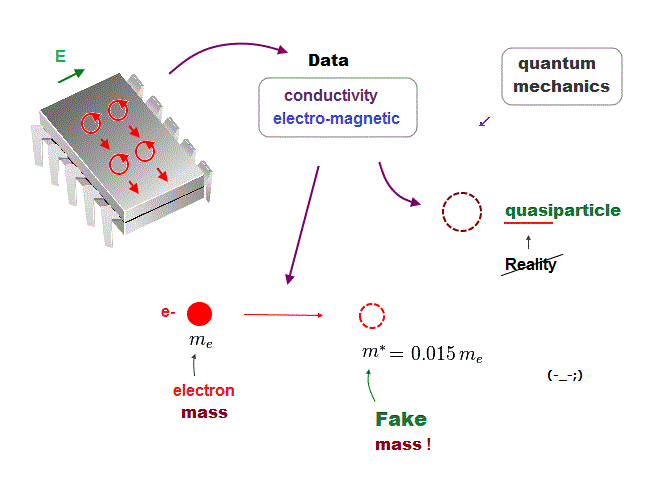In the present quantum mechanics, even when we get some new experimental data, we cannot use them to clarify real mechanisms !

Instead, quantum mechanics forces us to use these data to create artificial quasiparticle, effective mass ..  So our science stops its progress.

This is a very serious problem.  We must address it immediately not to increase more educational victims under false science.

## Only planetary model can keep two basic postulates.

### [ Two basic axioms: Coulomb and de Broglie wave are valid only in classical orbits. ]

(Fig.13)  Coulomb energy conservation and an integer times de Broglie wavelength.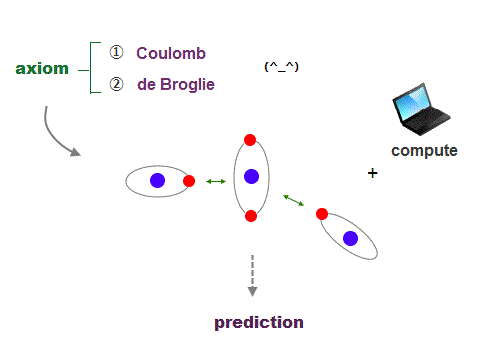It's quite natural that if we keep basic postulates, we can predict any physical values ( with the help of modern computer ).

These two postulates are Coulomb energy conservation ( in any electrons' positions ) and an integer times de Broglie wavelength generating Pauli force.

There is No way other than computing each Coulomb force and changing electrons' position in orthodox way to keep the single common ground state energy.

Of course, to obey an integer times de Broglie wave rule, this computing method using concrete orbits is the only one.

So the first thing for researchers to do is determine the more precise electronic motion model step by step, comparing experiments and the model's prediction.

I'm sure this realistic model based on two postulates will contribute to curing fatal diseases such as cancer, ALS, dementia someday.2016/6/24 updated. Feel free to link to this site.